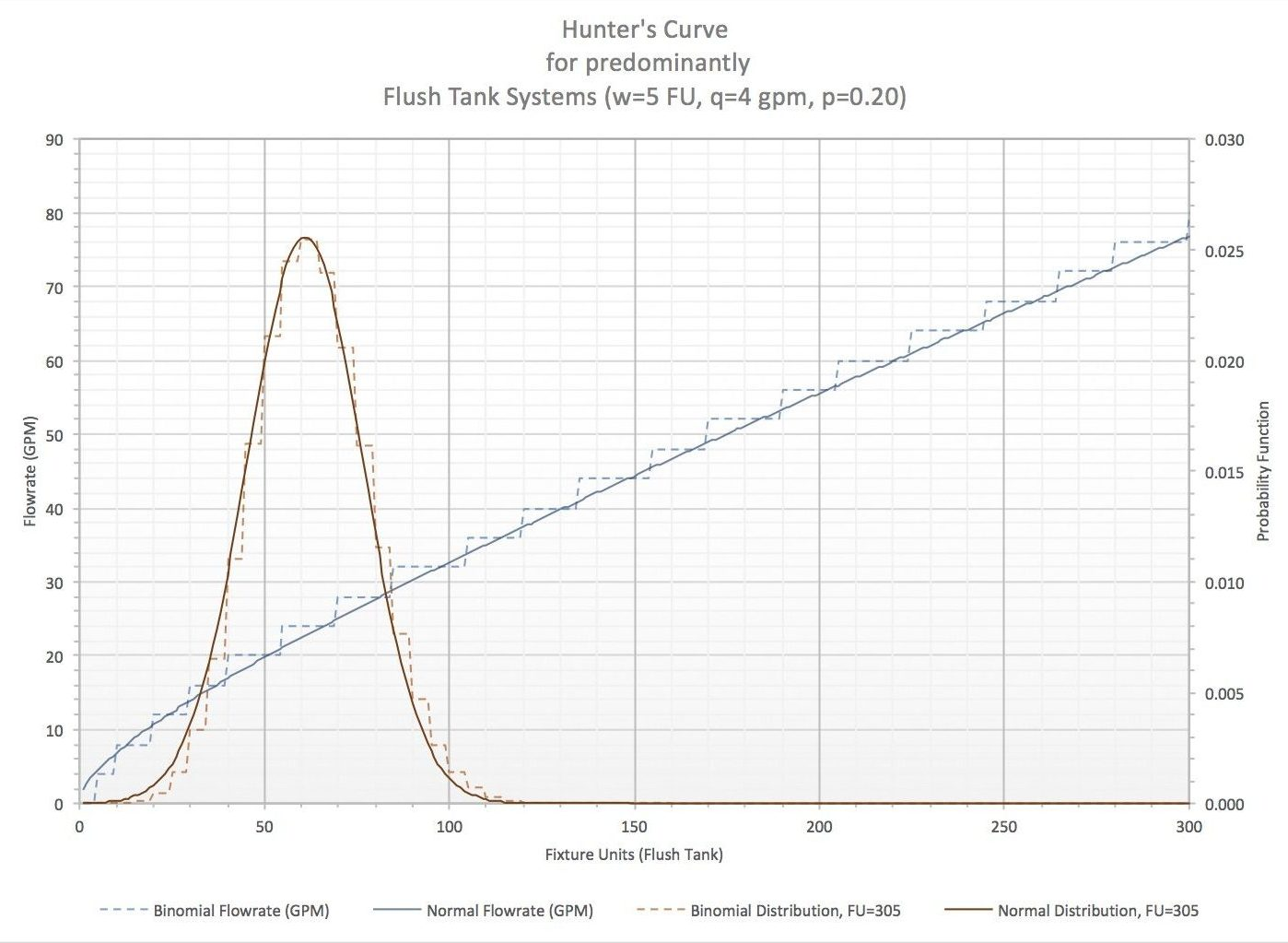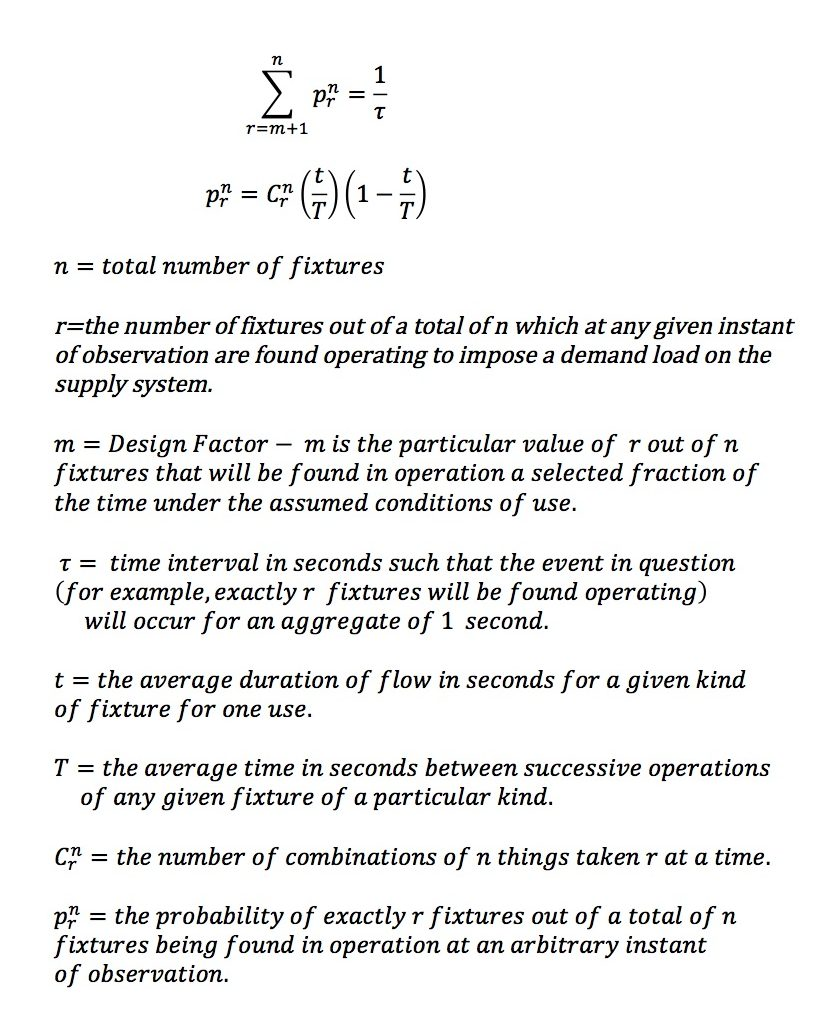# Tag Archives: plumbingfixtures

## Calculating Hunter’s Curve using ExcelHave you ever wondered how to convert from fixture units to flowrate? A curve fit on the code tables will not work satisfactorily! I have tried that myself. Here is the method as it was developed by Roy B. Hunter back in 1940. But don’t let this year set you back because even today’s code use his approach to estimate water flowrate demands for buildings.

Hunter’s approach to solve this engineering problem was statistical. This is the reason that a simple curve fit wont’t work. Hunter used a binomial distribution to estimate the probability that a given number of fixtures, m, out of a total of n, will be operating at an arbitrary instant of observation.

The equation he developed is as follows:Hunter used a 1/tau value of 0.01 which means that the probability of m fixtures being open is 99% or greater.

Because the curve was used for different kinds of plumbing fixtures, a fixture weighting factor (w) had to be incorporated. In systems with predominantly flush tanks, the weight for a Flush Tank w=5, for Flush Valves w=10. To covert from fixture units to fixture number the following equation is used, n=FU/W.

In order to calculate the demand in gallons per minute, a factor q equal to the average volume rate of flow, in gallons per minute, to or from the plumbing fixture during actual operation is used. Again, in the case of flush tanks systems q=4 gpm, for flush valve systems q=27 gpm. The demand flowrate (GPM) is the product m x q.

Although Hunter’s formulation seems complicated, it is relatively easy to calculate using excel. Excel has built in statistical functions to calculate the binomial probability function. In order to calculate the flow rate according to the binomial probability function the following equation must be entered into a cell.

[GPM]=BINOM.INV(FU/w,t/T,1-TAU)*q

Because the binomial probability function works with discrete values of fixtures (ie. 1,2,3,4,5,etc.), using fixture unit weights will lead to a steeped probability function as it can be depicted in the stepped bell shape and stepped demand flowrate curve shown above.

The Binomial Probability function can be converted to a continuous probability function using the normal probability. In this case the mean of the normal probability is nq and the variance is nq*p(1-p) with p=t/T. The following equation was used to calculate the smooth Hunter’s curve above.

[GPM]=NORMAL.INV(1-tau,FU/W*q,SQR(FU/W*q*(t/T)*(1-(t/T)))*q

Research by Hunter and other’s determined that the values for t and T depending on the predominant fixture type. For Flush Tank systems t=60, T=300 (p=0.20); for Flush Valve systems t=3 and T=300 (p=.01).  In this regard, current codes do little to specify these parameters that are highly dependent on the building classification and the characteristics of the fixtures used. It is my personal opinion that International codes should provide guidelines on which of the two probability functions to use, and prescribe a value for the probability (1-tau). International codes should also prescribe the values of t and q based on fixture characteristics and values of T based on building classification.